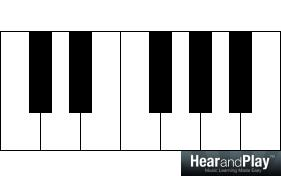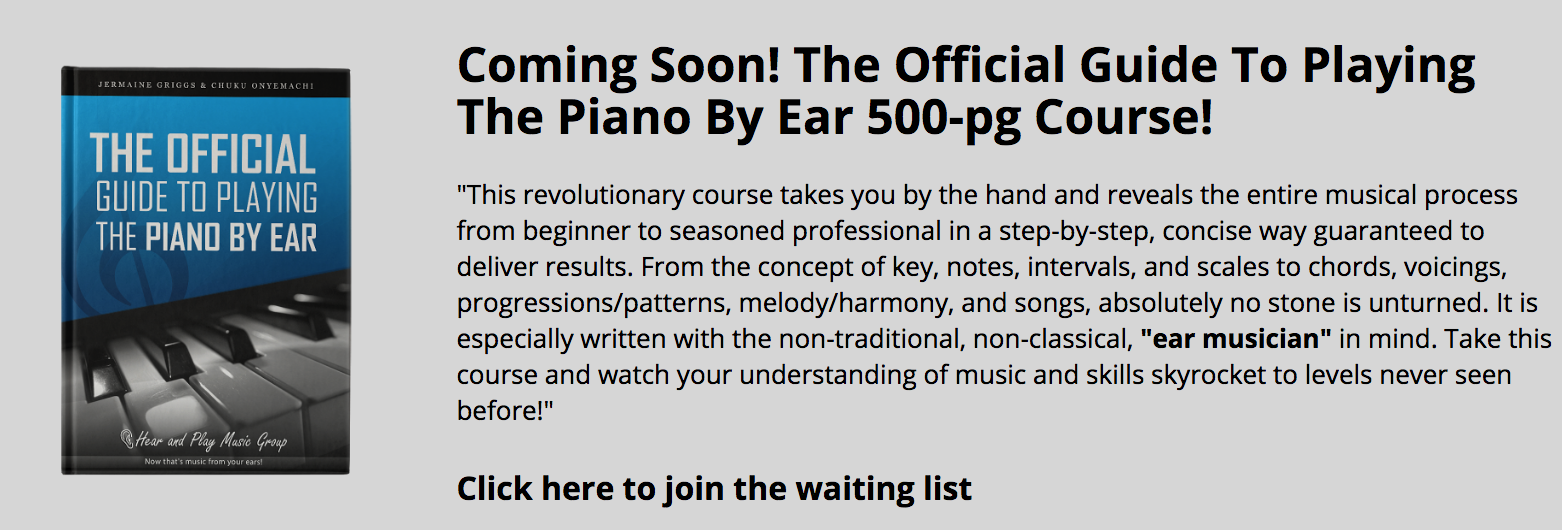• # Forming Seventh Chords Using Diatonic Dissonant IntervalsIntervals are used as building blocks in chord construction. This makes them harmonic devices that anyone desiring to build chords can depend on. Chords, irrespective of their quantity (or “width,” e.g. – 7th, 9th, 11th, 13th, etc.), can be broken down into intervals. These intervals are known as intervallic components. Today’s lesson focuses on dissonant intervals in particular and their role in building seventh chords.It can be broken down into:
C-E (Major third)C-G (Perfect fifth)In a previous post, The Relationship Between Dissonant Intervals, we covered dissonant intervals thoroughly and established the relationship between sevenths and seconds. In this article, we’ll focus more on how we can use these intervals in chord construction.

## TERTIAN HARMONY

A chord is basically a combination of notes. Here’s the C major chord again:The notes of a chord (like C, E and G in the example above) are called chord tones. This chord is a product of tertian harmony. In tertian harmony, chord tones are stacked in thirds.

For example, if we stack thirds from the major scale of C, we’ll have a triad:The triad is built off the first, third and fifth degrees of the scale.

If by happenstance or choice, we decide to add another third, we’ll have a seventh chord:The reason why it is called seventh chord is because:

1. It encompasses seven diatonic degrees – C, D, E, F, G, A and B
2. It is built off the first, third, fifth and SEVENTH degrees of the scale.

Now, we’ve found the seventh. In terms of chord construction, the seventh comes before the second in importance.

This doesn’t reduce the importance of the second. We’ll still find the second useful as a compound interval – ninth. The ninth is a bigger variation of the second.The interval above (C-D) can be played as:The occurrence of the seventh before the second (ninth) in tertian harmony will narrow our application to sevenths only. However, this does not exclude seconds from our study, considering that all sevenths are potential seconds.E.g. C-B (a seventh) above is a potential second. If inverted, C-B will become B-C (a second), as we learned in this past lesson.Therefore, ALL the sevenths we’ll explore in this course are potential seconds.

## SEVENTHS

There are two interval qualities of sevenths, the major seventh and the minor seventh. If you are not familiar with these seventh qualities, don’t worry. I’ll show you, step by step, how you can form these intervals using the Interval Shrinking Technique.

The Interval Shrinking Technique is the reduction of the size of an interval by raising the lower (bass) note or lowering the higher (melody) note using a given melodic progression. Let’s look at the two approaches to this.

Approach #1 – Raising the lower (bass) note.

Using the octave of C:…raising the lower C will yield D♭-C.This gives you D♭-C (an interval that is less than an octave).

Approach #2 – Lowering the higher note.

Using the octave of C:…lowering the higher C will yield C-B.This gives you C-B (another interval that is less than an octave).

Note:
Interval Shrinking Technique can also yield the diminished octave. The following intervals are NOT Major Sevenths even though they sound the same.

For example, using the first approach, we can raise the lower note to yield C♯-C:Using the second approach, we can lower the higher note to yield C-C♭:There are musical similarities to the major seventh and the diminished octave. If you want to know the similarities and differences between major sevenths and diminished octaves, I’ll recommend our upcoming HearandPlay 130 – Intervals course for you.

### THE BETTER APPROACH TO OCTAVE SHRINKING

Now that we’ve covered the Octave Shrinking Technique using two distinct approaches, let me show you the better approach and also explain why.

The First Approach gave us a D♭ Major 7th interval:The Second Approach yielded a C Major 7th interval:Each approach shrinks an octave and gives us a major seventh interval. However, the second approach is preferable. This is because it gives us a major seventh on the given key (C Major) as opposed to the first approach that gave us a major seventh on a different key (D♭ Major). Therefore, we’ll shrink the octave In this lesson by lowering the higher note (using the second approach).

## SEVENTH QUALITIES

Diatonic dissonant intervals have two seventh qualities – major and minor. We can derive each quality of seventh using the octave shrinking technique. It’s easier than it sounds.

### Major Seventh Intervals

Shrinking of the octave by a semitone progression (or half step) will yield a major seventh interval.
Therefore,

Producing the C major seventh interval is as easy as shrinking an Octave of C by a semitone progression (half step):Forming a D♭ major seventh interval is as easy as shrinking an Octave of D♭ by a semitone progression:D Major seventh interval is as easy as shrinking an Octave of D by a semitone progression.E♭ Major seventh interval is as easy as shrinking an Octave of E♭ by a semitone progression.If we go on and on, we’ll have major seventh intervals on ALL the keys with little to no effort.

### Minor Seventh Intervals

Shrinking of the octave by a wholetone progression will yield a minor seventh interval.

Therefore…

C Minor seventh interval is as easy as shrinking an Octave of C by a wholetone progression.C♯ Minor seventh interval is as easy as shrinking an Octave of C♯ by a wholetone progression.D Minor seventh interval is as easy as shrinking an Octave of D by a wholetone progression.D♯ Minor seventh interval is as easy as shrinking an Octave of D♯ by a wholetone progression.That’s how we can derive minor seventh intervals on ALL the keys with little to no effort.

## SEVENTH CHORDS FROM SEVENTH INTERVALS

Right from the beginning of this lesson, I’ve always emphasized that intervals are components of chords. Now that we have learned two diatonic dissonant intervals – major seventh and minor seventh, let’s get into chord formation using these intervals.

### Chord Formation #1 – Major Seventh Chord

Using the formula below, we can derive the major seventh chord on any key.

Major Triad + Major 7th interval = Major 7th Chord.

Example 1+ C Major Seventh interval (C-B)= C Major Seventh Chord (C-E-G-B)Example 2+ B Major Seventh interval (B-A♯)= B Major Seventh Chord (B-D♯-F♯-A♯)Example 3+ A Major Seventh interval (A-G♯)= A Major Seventh Chord (A-C♯-E-G♯)### Chord Formation #2 – Minor Seventh Chord

Using the formula below, we can derive the minor seventh chord on any key.

Minor Triad + Minor 7th interval = Minor 7th Chord.

Example 1+ C Minor Seventh interval (C-B♭)= C Minor Seventh Chord (C-E♭-G-B♭)Example 2+ B Minor Seventh interval (B-A)= B Minor Seventh Chord (B-D-F♯-A)Example 3+ A Minor Seventh interval (A-G)= A Minor Seventh Chord (A-C-E-G)### Chord Formation #3 – Dominant Seventh Chord

Using the formula below, we can derive the dominant seventh chord on any key.

Major Triad + Minor 7th interval = Dominant 7th Chord.

Example 1+ C Minor Seventh interval (C-B♭)= C Dominant Seventh Chord (C-E-G-B♭)Example 2+ B Minor Seventh interval (B-A) =B Dominant Seventh Chord (B-D♯-F♯-A)Example 3+ A Minor Seventh interval (A-G)= A Dominant Seventh Chord (A-C♯-E-G)## Final Words

From this post, you’ve learned how to play major and minor seventh intervals and how to effortlessly use them in the construction of chords. I want to recommend that you practice chord formation in all the keys on your own.

P.S.

All these seventh chords are dissonant. Their dissonant property is inherited from diatonic dissonant intervals. Practically, the major and minor seventh have tolerable dissonance while the dominant seventh chord sounds extremely dissonant. In subsequent articles, you’ll find out why.

I’ll see you next time.

The following two tabs change content below.#### Chuku Onyemachi

Head of Education at HearandPlay Music Group
Onyemachi "Onye" Chuku (aka - "Dr. Pokey") is a Nigerian musicologist, pianist, and author. Inspired by his role model (Jermaine Griggs) who has become his mentor, what he started off as teaching musicians in his Aba-Nigeria neighborhood in April 2005 eventually morphed into an international career that has helped hundreds of thousands of musicians all around the world. Onye lives in Dubai and is currently the Head of Education at HearandPlay Music Group and the music consultant of the Gospel Music Training Center, all in California, USA.#### Latest posts by Chuku Onyemachi (see all)